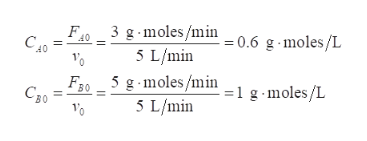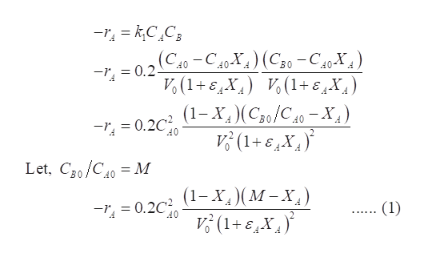# Effect of ε value on conversion in PFR reactor: Consider the following similar reaction stoichiometries (assume gas phase reaction):Case a:  A +  B  →  CCase b:  A +  B  →  2CCase c:  A +  B  →  4CUse the same reaction rate law of –rA= kCACBfor all three cases, with k = 0.2 L/gr mole-min.  The reactor feeds are FA0= 3 gr mole/min and FB0= 5 gr mole/min; volumetric flow rate is 5 L/min.  Also, there are no inerts present, and there is no C in the feed.  Using a single-tube plug flow reactor of dimensions length L = 6 m and ID = 0.1 m, prepare the integral form of the PFR reactor design equation(with rate law and constants substituted into the equation) for each case.  Then, use your calculator, numerical integration, or MATLAB to solve the differential equation dXA/dV for each case.  Using the output of XAversus V (the cumulative volume as one travels along the reaction length) for all three cases, create a single plot of XA(y-axis) versus V (X-axis), and label each of the three curves on the plot with its respective value of ε. Which value of ε (which case) gives the highest value of conversion?

Question
146 views
1. Effect of ε value on conversion in PFR reactor: Consider the following similar reaction stoichiometries (assume gas phase reaction):

Case a:  A +  B  →  C

Case b:  A +  B  →  2C

Case c:  A +  B  →  4C

Use the same reaction rate law of –rA= kCACBfor all three cases, with k = 0.2 L/gr mole-min.  The reactor feeds are FA0= 3 gr mole/min and FB0= 5 gr mole/min; volumetric flow rate is 5 L/min.  Also, there are no inerts present, and there is no C in the feed.  Using a single-tube plug flow reactor of dimensions length L = 6 m and ID = 0.1 m, prepare the integral form of the PFR reactor design equation(with rate law and constants substituted into the equation) for each case.  Then, use your calculator, numerical integration, or MATLAB to solve the differential equation dXA/dV for each case.  Using the output of XAversus V (the cumulative volume as one travels along the reaction length) for all three cases, create a single plot of XA(y-axis) versus V (X-axis), and label each of the three curves on the plot with its respective value of ε. Which value of ε (which case) gives the highest value of conversion?

check_circle

Step 1

The given information are:

Case a: A+B→C

Case b: A+B→2C

Case c: A+B→4C

-rA = kCACB for all the cases and

k = 0.2 L/(g.mol-min)

FA0= 3 g-mol/min

FB0= 5 g-mol/min

v0 = 5 L/min

For single-tube plug flow reactor,

L = 6m

ID = 0.1 m

No inerts or C is present in the feed.

Initial volume of the reactor is calculated as:

Step 2

From the given data, calculate the values of CA0 and CB0 as:help_outlineImage Transcriptionclose3 g moles/min 5 L/min F40 CA0 g moles/L = 0.6 5 g-moles/min 5 L/min F30 C50 -moles/L fullscreen
Step 3

In this rate law, CA and CB can be written in terms of XA, fractional conversion, initial concentration of reactants, CA0 and CB0, and &e...help_outlineImage Transcriptionclose(C40-C40X) (C0-C40-X) 0.2 Vo(1+EX) (1+6X) (1-X,)(Cso/C40-X, V(1+6X 0.2 40 Let, Cg0/C0M (1-X) (M-X V(1+EX 0.2 (1) 40 fullscreen

### Want to see the full answer?

See Solution

#### Want to see this answer and more?

Solutions are written by subject experts who are available 24/7. Questions are typically answered within 1 hour.*

See Solution
*Response times may vary by subject and question.
Tagged in

### Chemical Technology